Next: Free Expansion of Gas Up: Classical Thermodynamics Previous: Gibbs Free Energy

# General Relation Between Specific Heats

Consider a general homogeneous substance (not necessarily a gas) whose volume,, is the only relevant external parameter. Let us find the general relationship between this substance's molar specific heat at constant volume,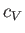, and its molar specific heat at constant pressure,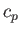.

The heat capacity at constant volume is given by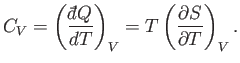(6.120)

Likewise, the heat capacity at constant pressure is written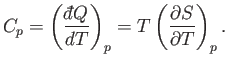(6.121)

Experimentally, the parameters that are most easily controlled are the temperature,, and the pressure,. Let us consider these as the independent variables. Thus,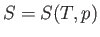, which implies that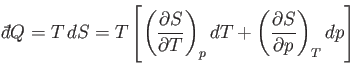(6.122)

in an infinitesimal quasi-static process in which an amount of heat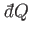is absorbed. It follows from Equation (6.121) that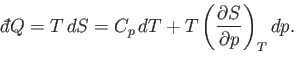(6.123)

Suppose that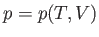. The previous equation can be written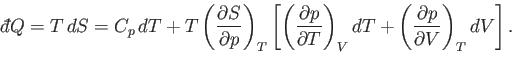(6.124)

At constant volume,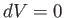. Hence, Equation (6.120) gives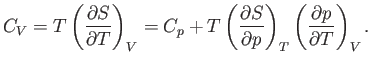(6.125)

This is the general relationship between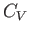and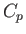. Unfortunately, it contains quantities on the right-hand side that are not readily measurable.

Consider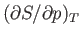. According to the Maxwell relation (6.119),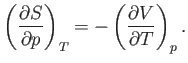(6.126)

Now, the quantity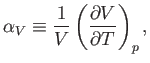(6.127)

which is known as the volume coefficient of expansion, is easily measured experimentally. Hence, it is convenient to make the substitution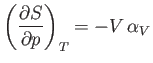(6.128)

in Equation (6.125).

Consider the quantity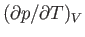. Writing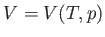, we obtain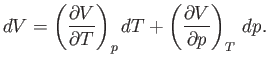(6.129)

At constant volume,, so we obtain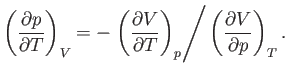(6.130)

The (usually positive) quantity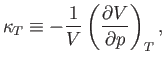(6.131)

which is known as the isothermal compressibility, is easily measured experimentally. Hence, it is convenient to make the substitution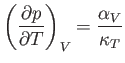(6.132)

in Equation (6.125). It follows that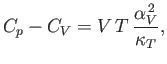(6.133)

and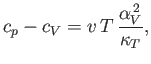(6.134)

where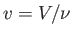is the molar volume.

As an example, consider an ideal gas, for which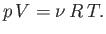(6.135)

At constant, we have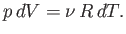(6.136)

Hence,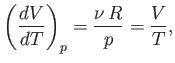(6.137)

and the expansion coefficient defined in Equation (6.127) becomes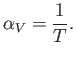(6.138)

At constant, we have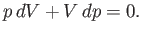(6.139)

Hence,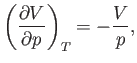(6.140)

and the compressibility defined in Equation (6.131) becomes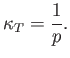(6.141)

Finally, the molar volume of an ideal gas is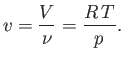(6.142)

Hence, Equations (6.134), (6.138), (6.141), and (6.142) yield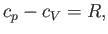(6.143)

which is identical to Equation (6.39).Next: Free Expansion of Gas Up: Classical Thermodynamics Previous: Gibbs Free Energy
Richard Fitzpatrick 2016-01-25